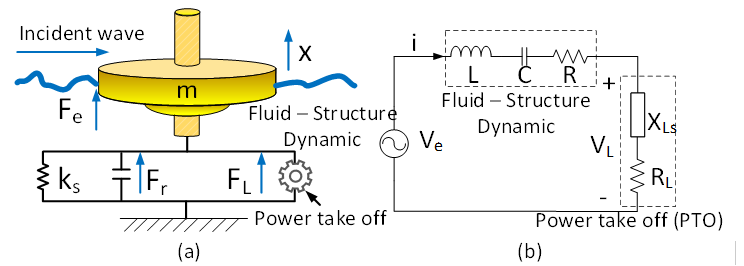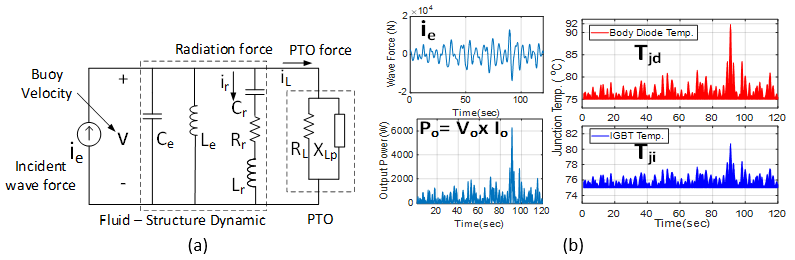LIBRARY

# A Wave-to-Wire Equivalent Circuit for Irregular (Regular) Wave

Year: 2018 | Author: Chien-An Chen | Paper: D2.4Fig. 1. (a) A wave energy converter (WEC) with submerged body, and power take-off and (b) its electric analogy: a conventional monochromatic (single-frequency) RLC circuit model (forces represented by voltages).
A wave-to-wire (W2W) model from a wave energy converter (WEC), a mechanical-electrical composite system which converts ocean wave energy to electrical power, is shown in Fig. 1. The WEC system starts from a buoy-wave interaction coupling with a rotational mechanical system. A mechanical motion rectifier then drives the permanent magnet synchronous generator, which delivers energy to a power electronics converter.

Through approximation and circuit synthesis methods, wave-buoy behavior can be linearized as an RLC circuit network in Fig. 2 (a). A general methodology is shown converting a multidisciplinary system into electrical components, which can be an aid to electrical designers in the wave energy area.

An electric-based W2W model is presented and applied in a reliability analysis of power electronics components. Based on an equivalent circuit, the system output power capability and thermal stress can thus be analyzed in the time domain under an irregular wave condition in Fig. 2 (b).Fig. 2. (a) Equivalent circuit of the WEC, and (b) testing of equivalent circuit in time domain.